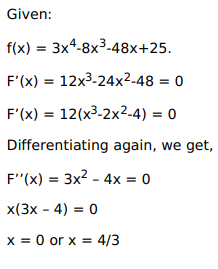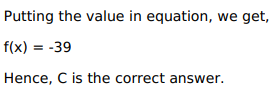# Solve this following

Question:

Mark $(\sqrt{)}$ against the correct answer in the following:

The minimum value of $f(x)=3 x^{4}-8 x^{3}-48 x+25$ on $[0,3]$ is

A. 16

B. 25

C. $-39$

D. none of these

Solution: